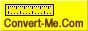## How do I answer this maths problem ?

Try to post your problem here, there is a chance, someone clever will help you.
Forum rules
Dear convert-me.com forum visitors,

Our forum has been available for many years. In September 2014 we decided to switch it to read-only mode. Month after month we saw less posts with questions and answers from real people and more spam posts. We were spending more and more resources cleaning the spam until there were less them 1 legitimate message per 100 spam posts. Then we decided it's time to stop.

All the posts in the forum will be available and searchable. We understand there are a lot of useful information and we aren't going to remove anything. As for the new questions, you can always ask them on convert-me.com FaceBook page

Thank you for being with us and sorry for any inconveniences this could caused.

### How do I answer this maths problem ?

How do I answer this maths problem on vectors in component form?
Could someone please solve and explainl.
The question is:

If a = 2i + 3j & b=3i - 4j & c = 2i + j then find x and y such that c= xa + yb.
powelstag

Posts: 4
Joined: Fri Feb 12, 2010 12:56 pm

### Re: How do I answer this maths problem ?

powelstag wrote:How do I answer this maths problem on vectors in component form?
Could someone please solve and explainl.
The question is:

If a = 2i + 3j & b=3i - 4j & c = 2i + j then find x and y such that c= xa + yb.

The i and j components are independent and can be calculated separately:
The i component: 2 = 2x + 3y
The j component: 1 = 3x + 4y

From the first equation we get x = (2-3y)/2, and then 1 = 3/2(2-3y) + 4y, or 1 = 3 - 4.5y + 4y, or 0.5 y = 2, i.e. y = 4
Then x = (2-3*4)/2 = -5
Convert-Me.Com - Interactive Units Conversion
http://www.convert-me.com/convert-me.com

Posts: 402
Joined: Fri Sep 05, 2003 4:50 pm

• List of all units you can convert online
• Metric conversion
• Convert grams to cups
• Grams to milliliters
• Imperial vs US Customary
• History of measurement